### Home > A2C > Chapter 6 > Lesson 6.1.1 > Problem6-14

6-14.
1. Jamilla collected data comparing the weight and cost of pieces of sterling silver jewelry. Her data is listed as (weight in ounces, cost in dollars): (5, 44.00), (8.5, 78.50), (12, 112.00), (10, 93.00), (7, 63.50), (9, 83.20). 6-14 HW eTool (Desmos). Homework Help ✎

1. Plot the data on a set of axes.

2. Use a ruler to draw a line that best approximates the data.

3. Determine the equation of the line of best fit drawn in (b).

4. Use your equation to predict the cost of a 50-ounce silver bracelet.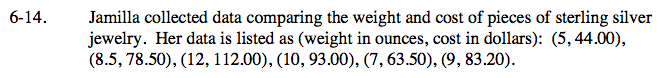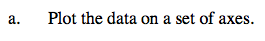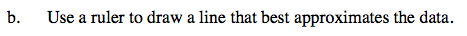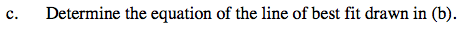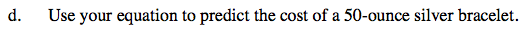Use eTool below to solve the parts of this problem.
Click the link at right for the full version of the eTool: A2C 6-14 HW eTool